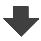Search:

# Examples of electrolysis

## Exercise 3

Electrolysis of a sodium iodide solution is done between (inert) graphite electrodes.
Give the formulas of the ions which are in presence !

Ions : $Na^+$ ; $I^{-}$ from dissolution and dissociation of sodium iodide $H^{+}$ ; $OH^-$ from the partial dissociation of water.

Look for the strongest oxidant - for the strongest reductant !

$H^{+}$ is the strongest oxidant; $I^{-}$ is the strongest reductant.

Write the equations of the reactions occuring at the electrodes !

Cathode: $2H^{+}$ $+$ $2e^-$ $\longrightarrow$ $H_2$ (reduction) Anode: $2I^-$ $-$ $2e^-$ $\longrightarrow$ $I_2$ (oxidation)

What is observed?

At the cathode: Release of hydrogen, a colourless gas. At the anode: Formation of brownish iodine soluble in the presence of iodide.